# Calculate Heat of Hydration

Calculate Heat of Hydration

Heat of Hydration
Heat evolved by chemical reactions with water, such as that evolved during the setting and hardening of Portland cement, or the difference between the heat of solution of dry cement and that of partially hydrated cement

Formula to Determine Heat of Hydration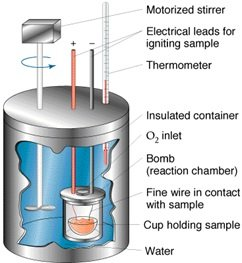H = H1H2 – 0.4(th – 25.0)
Where
H = heat of hydration of ignited cement, kJ/kg,
H1= heat of solution of dry cement
H2 = heat of solution of partially hydrated sample
th=  final calorimeter temperature at end of determination on partially hydrated sample, °C.

ASTM Code For Heat of Hydration
ASTM C-186  is used to determine the heat of hydration.
“Standard Test Method for Heat of Hydration of Hydraulic Cement”

Apparatus Used
Calorimeter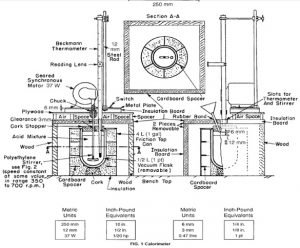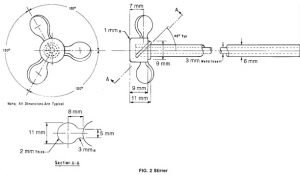Parts of Calorimeter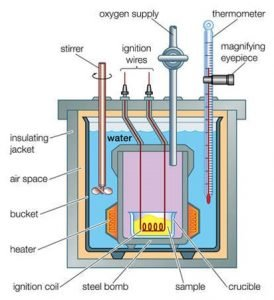Procedure

• Determine the Heat of Solution of the dry cement sample

To determine the heat capacity of the system (that is, the number of joules or calories required to raise the temperature of the calorimeter and contents 1°C), measure the corrected temperature rise obtained by dissolving 7 g of ignited ZnO in the specified acid mixture (see 6.2-6.7). 6.2 Transfer approximately 400 g of the 2.00 N HNO3, which has been cooled to the temperature indicated by the lower range of the Beckmann thermometer (ordinarily about 4 to 5°C below room temperature), into the vacuum jar, add 8.0 mL of HF (sp gr 1.15), weigh, and add sufficient additional 2.00 N HNO3 to bring the total weight of the solution to 425.0 g. Then, assemble the calorimeter and start the stirring motor. Take care that the stirrer blades or shaft do not touch the thermometer, the sides or bottom of the jar, or the cork stopper. The lower end of the funnel stem shall extend approximately 6 mm (1⁄4 in.) below the lower surface of the stopper and at least 12 mm (1⁄2 in.) above the level of the liquid. The upper end of the bulb of the Beckmann thermometer shall be at least 38 mm (11⁄2 in.) below the surface of the liquid. Place it at the same depth in all determinations. After an initial stirring period of at least 20 min to allow the temperature of the system to become uniform, record the temperature of the room to the nearest 0.1°C, the temperature of the acid to the nearest 0.001°C, record the time, and then immediately introduce the prepared ZnO through the funnel at a uniform rate . Complete the itroduction of the ZnO in not less than 1 or more than 2 min. Brush any ZnO clinging to the funnel stem into the acid mixture by means of a small “camel’s-hair” brush.
Read the temperature, to the nearest 0.001°C, at 20 min and again at 40 min after beginning the introduction of the sample. The first 20-min period is the uncorrected temperature rise, which covers the solution period. The second 20-min period is the rating period, and the temperature difference between the 20 and 40-min readings is the correction to be added to or subtracted from the uncorrected temperature rise, according to whether the calorimeter temperature rises or falls during the rating period.

• Determine the Heat of Solution of the dry cement sample by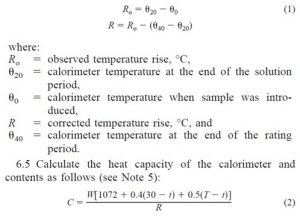where:
C = heat capacity, kJ/°C,
W = mass of cement, 3g,
t = final temperature of the calorimeter, °C (u20 plus temperature, °C, at which the Beckmann thermometer reading is zero),
T = temperature of the ZnO (room temperature), °C, when introduced into the calorimeter, and
R = corrected temperature rise, °C.

• Determine the Heat of Solution of partially hydrate sample by the same procedure as for the dry cement described above, but use a 4.18 +- 0.05-g calorimetric sample of the partially hydrated cement, weighed to the nearest 0.001 g . Calculate the results on the ignited basis.
• Loss on Ignition:

Portland Cement—Immediately before and after the calorimetric sample is being weighed out, weigh a sample of similar amount into a platinum crucible for determination of loss on ignition, the value to be used being the average of the two determinations. Ignite the dry cement at 950 +- 50°C for at least 11⁄2 h or to constant mass. Immediately place the crucible containing the sample in a desiccator and allow to cool to room temperature; then quickly weigh the crucible. When determining the loss on ignition of the hydrated cement, first dry the weighed sample in an oven at 100 to 110°C for 1 h; then place the sample in a muffle furnace at 950 +- 50°C overnight, or  bring to constant mass. Reduce the mass of the cement sample that was introduced into the calorimeter to the ignited mass basis for use in the final calculations as follows:
Wi  = (A/B) / W

Where
Wi = mass of calorimetric sample, on ignited basis, g,
A = mass of ignited sample, g,
B = mass of sample before ignition, g, and
W = mass of calorimetric sample, g

• Blended Hydraulic Cements—In addition to the procedures described above, determine the loss on ignition by the reference method given in Test Methods C 114 for Portland blast-furnace slag cement and slag cement.
• Determine the SO3 content by the reference method given in Test Methods C 114 (see Note 6). Also determine the SO3 content of a portion of the same cement that has not been ignited, using the same procedure.
• Calculate the percentage of mass gain from sulfide sulfur as follows:

G = 0.8 (S1 – S2)

where:
G = percent mass gain in ignited sample,
S1 = SO3 determined on ignited sample, and
S2 = SO3 determined on unignited sample.
0.8 = molecular weight ratio of 4(0)/SO3

Calculate the mass of the dry calorimetric sample on the ignited basis as follows: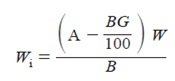where:
Wi = mass of dry calorimetric sample, on ignited basis, g,
A = mass of ignited dry sample, g,
B = mass of dry sample before ignition, g,
G = percentage mass gain from sulfide sulfur, and
W = mass of dry calorimetric sample, g.

• Calculate the mass of the partially hydrated calorimetric sample on the ignited basis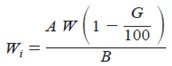where:
Wi = mass of calorimetric sample, on ignited basis, g,
A = mass of partially hydrated sample after ignition, g,
B= mass of partially hydrated sample before ignition, g
G = percentage mass gain from sulfide sulfur, and
W = mass of partially hydrated calorimetric sample, g.

Calculations of Heat of Hydration of Cement on next Post. Click Here to See.

Calculate Heat of Hydration, Calculate Heat of Hydration, Calculate Heat of Hydration, Calculate Heat of Hydration, Calculate Heat of Hydration, Calculate Heat of Hydration, Calculate Heat of Hydration, Calculate Heat of Hydration, Calculate Heat of Hydration, Calculate Heat of Hydration, Calculate Heat of Hydration, Calculate Heat of Hydration, Calculate Heat of Hydration, Calculate Heat of Hydration, Calculate Heat of Hydration, Calculate Heat of Hydration, Calculate Heat of Hydration, Calculate Heat of Hydration, Calculate Heat of Hydration, Calculate Heat of Hydration, Calculate Heat of Hydration, Calculate Heat of Hydration, Calculate Heat of Hydration, Calculate Heat of Hydration, Calculate Heat of Hydration, Calculate Heat of Hydration, Calculate Heat of Hydration, Calculate Heat of Hydration, Calculate Heat of Hydration